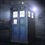# A nice but easy proof!

Prove that the value of, $\sum_{n=1}^{n}\dfrac{1}{n^2}<2$.Feel free to post your innovative methods! I have posted mine below!4 years, 9 months ago

This discussion board is a place to discuss our Daily Challenges and the math and science related to those challenges. Explanations are more than just a solution — they should explain the steps and thinking strategies that you used to obtain the solution. Comments should further the discussion of math and science.

When posting on Brilliant:

• Use the emojis to react to an explanation, whether you're congratulating a job well done , or just really confused .
• Ask specific questions about the challenge or the steps in somebody's explanation. Well-posed questions can add a lot to the discussion, but posting "I don't understand!" doesn't help anyone.
• Try to contribute something new to the discussion, whether it is an extension, generalization or other idea related to the challenge.

MarkdownAppears as
*italics* or _italics_ italics
**bold** or __bold__ bold
- bulleted- list
• bulleted
• list
1. numbered2. list
1. numbered
2. list
Note: you must add a full line of space before and after lists for them to show up correctly
paragraph 1paragraph 2

paragraph 1

paragraph 2

[example link](https://brilliant.org)example link
> This is a quote
This is a quote
    # I indented these lines
# 4 spaces, and now they show
# up as a code block.

print "hello world"
# I indented these lines
# 4 spaces, and now they show
# up as a code block.

print "hello world"
MathAppears as
Remember to wrap math in $$ ... $$ or $ ... $ to ensure proper formatting.
2 \times 3 $2 \times 3$
2^{34} $2^{34}$
a_{i-1} $a_{i-1}$
\frac{2}{3} $\frac{2}{3}$
\sqrt{2} $\sqrt{2}$
\sum_{i=1}^3 $\sum_{i=1}^3$
\sin \theta $\sin \theta$
\boxed{123} $\boxed{123}$

Sort by:

Isn't this reimann zeta(2)? Then the value will be 1.64 only.

- 4 years, 8 months ago

Yes,it is but that isn't the intended proof,or else you will have to prove how you found the value of $\zeta{(2)}$.

- 4 years, 8 months ago

Oh so that's why proof! Btw my method was just like yours

- 4 years, 8 months ago

from the basel problem we get $\sum_{n=1}^\infty (\frac{1}{n^2}) = \frac{\pi^2}{6}<2$. although this is probably not intended, but still works as a good proof. we prove the basel proble: Euler's original derivation of the value $\frac{π^2}{6}$ essentially extended observations about finite polynomials and assumed that these same properties hold true for infinite series.

recall the Taylor series expansion of the sine function

$\sin(x) = x - \frac{x^3}{3!} + \frac{x^5}{5!} - \frac{x^7}{7!} + \cdots.$ Dividing through by x, we have

$\frac{\sin(x)}{x} = 1 - \frac{x^2}{3!} + \frac{x^4}{5!} - \frac{x^6}{7!} + \cdots.$ Using the Weierstrass factorization theorem, it can also be shown that the left-hand side is the product of linear factors given by its roots, just as we do for finite polynomials

\begin{aligned} \frac{\sin(x)}{x} &= \left(1 - \frac{x}{\pi}\right)\left(1 + \frac{x}{\pi}\right)\left(1 - \frac{x}{2\pi}\right)\left(1 + \frac{x}{2\pi}\right)\left(1 - \frac{x}{3\pi}\right)\left(1 + \frac{x}{3\pi}\right) \cdots \\ &= \left(1 - \frac{x^2}{\pi^2}\right)\left(1 - \frac{x^2}{4\pi^2}\right)\left(1 - \frac{x^2}{9\pi^2}\right) \cdots. \end{aligned} If we formally multiply out this product and collect all the x2 terms (we are allowed to do so because of Newton's identities), we see that the x2 coefficient of sin(x)/x is

$-\left(\frac{1}{\pi^2} + \frac{1}{4\pi^2} + \frac{1}{9\pi^2} + \cdots \right) = -\frac{1}{\pi^2}\sum_{n=1}^{\infty}\frac{1}{n^2}.$ But from the original infinite series expansion of sin(x)/x, the coefficient of x2 is −1/(3!) = −1/6. These two coefficients must be equal; thus,

$-\frac{1}{6} = -\frac{1}{\pi^2}\sum_{n=1}^{\infty}\frac{1}{n^2}.$ Multiplying through both sides of this equation by $-\pi^2$ gives the sum of the reciprocals of the positive square integers.

$\sum_{n=1}^{\infty}\frac{1}{n^2} = \frac{\pi^2}{6}.$

- 4 years, 9 months ago

Thanks a lot for adding the proof!

- 4 years, 9 months ago

You are right,it wasn't intended but if you write this,you are expected to prove it too.

- 4 years, 9 months ago

fine

- 4 years, 9 months ago

We basically have to prove that,$\sum_{n=2}^{n}\dfrac{1}{n^2}<1$ $\text{Motivation of proof}:$,the first idea that struck me was the use of telescoping series,if i could write,$\dfrac{1}{n^2}<\text{something}$ and write,$\dfrac{1}{(n-1)^2}<\text{another something}$,and when we add the terms of $L.H.S$ we would get the required expression and when we add the terms of the $R.H.S$ we would get a telescoping series.The next thing that came to my mind was that,$n^2>(n)(n-1)$(we have taken the greater than sign as then when we take the reciprocal the sign would get reversed),since $\dfrac{1}{(n)(n-1)}=\dfrac{1}{n-1}-\dfrac{1}{n}$,and we would get a telescoping series like this, $\dfrac{1}{2^2}<\dfrac{1}{1}-\dfrac{1}{2}\\ \dfrac{1}{3^2}<\dfrac{1}{2}-\dfrac{1}{3}\\ .\\ .\\ .\\ \dfrac{1}{n^2}<\dfrac{1}{n-1}-\dfrac{1}{n}\\ \text{adding,we get}:\\ \sum_{n=2}^{n}\dfrac{1}{n^2}<1-\dfrac{1}{n}<1$.Hence proved.And done!

- 4 years, 9 months ago

From where you got this motivation ?

- 4 years, 9 months ago

I am sorry but i don't understand the meaning of your comment.Could you please explain?

- 4 years, 9 months ago

Simplification: Source of the question = ?

- 4 years, 9 months ago

- 4 years, 9 months ago

I like your motivations haha :P

- 4 years, 9 months ago

Well, yours is the simplest way. For the sake of mentioning, one can also write a proof by induction for this.

- 4 years, 9 months ago

How do you do a proof by induction (that is fundamentally different from his approach)?

Staff - 4 years, 9 months ago

@Calvin Lin Oops, although Induction is tempting at first look, it isn't the best way to go about. I haven't found an inductive proof yet 😕.

- 4 years, 9 months ago

Right. The inductive proof that I know, is to show that for $n \geq 2$,

$\sum_{i=1}^n \frac{1}{i^2 } < 2 - \frac{1}{n}.$

This is similar to what Adarsh did.

Staff - 4 years, 9 months ago